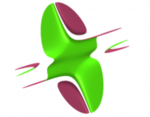# NameSurfer

programmeNameSurfer 0.2.1 setup for Windows 32-bit (for Mac and Linux packages see below).

## Crédits

NameSurfer has be developed at the ITBA University in Buenos Aires, Argentina in 2011.

## Contributeurs

DeveloperXin Wei Gou

With NameSurfer you can experience the relation between letters, words, formulas and forms, i. e. mathematics and art, in an interactive way. You can enter simple equations or words as for example your name. They will produce beautiful images, which are surfaces in space. Mathematically, the program visualizes real algebraic geometry in real-time. The surfaces shown are given by the zero set of a polynomial equation in 3 variables. The letters/words are transformed into algebraic equations.

With NameSurfer you can experience the relation between letters, words, formulas and forms, i. e. mathematics and art, in an interactive way. You can enter simple equations or words as for example your name. They will produce beautiful images, which are surfaces in space. Mathematically, the program visualizes real algebraic geometry in real-time. The surfaces shown are given by the zero set of a polynomial equation in 3 variables. The letters/words are transformed into algebraic equations. NameSurfer is an extension of the Java implementation
of Surfer 2012. It was developed at the ITBA University in Buenos Aires Argentina.